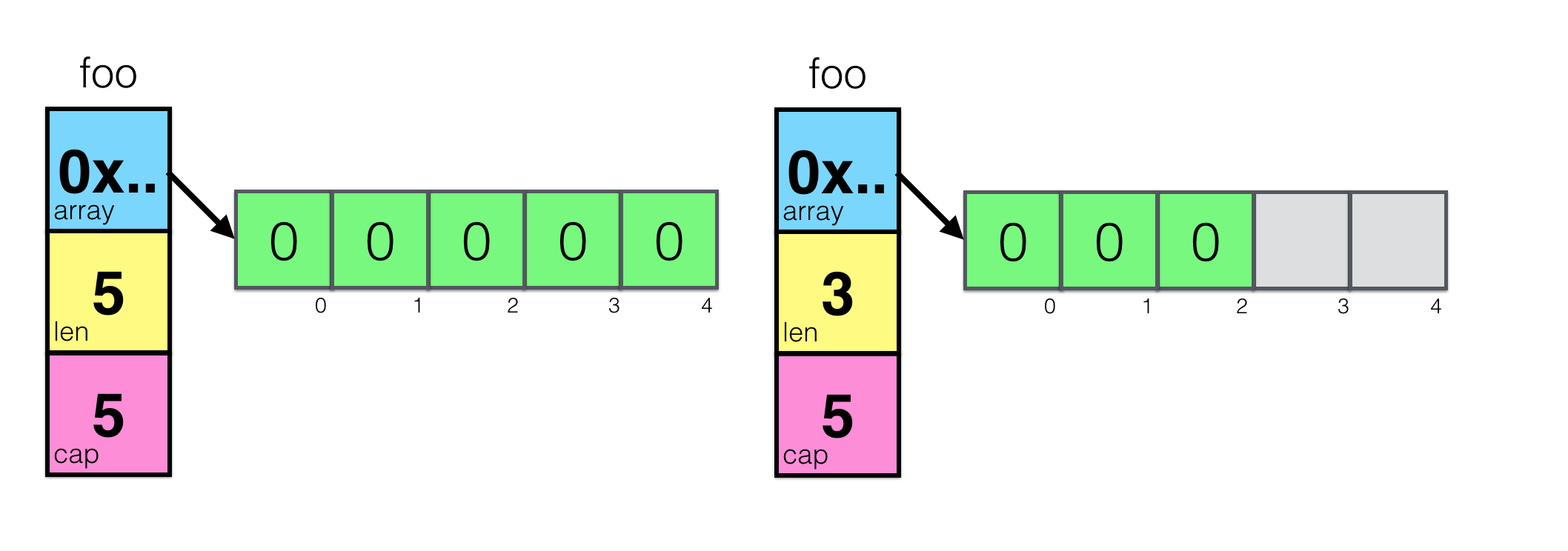## Some Details About Golang Slice

In Golang, there are two types of data:

One is an array with a fixed length, called Array, and the other is an array with an unlimited length, called Slice.

## Distinguish Between Array and Slice

The difference between Array and Slice is:

Array is of fixed length, and the length of Array is part of the type, so the length of Array cannot be changed, while the length of Slice can be changed.

Slice is of unlimited length and can be created using the `make` function.

`foo = make([]int, 5)` And Slice is just a data structure, there is a pointer inside, pointing to the first address of the array, you can use the `len` function to get the length of the Slice, and you can also use the `cap` function to get the capacity of the Slice.

The following will introduce some implementation details and characteristics of Slice in detail.

## Implementation and Characteristics of Slice

As mentioned above, Slice is actually a data structure, and there is a pointer inside, pointing to the first address of the array. Let’s take a simple look at the implementation of Slice:

Let’s first give a simple data structure to demonstrate the implementation of Slice:

 ``````1 2 3 4 5 `````` ``````type slice struct { array unsafe.Pointer len int cap int } ``````

We can see that the implementation of Slice is a structure, which includes three fields: The first field is a pointer to the underlying array, the second field is the length of the Slice, and the third field is the capacity of the Slice. When you initialize a Slice with a length of 5, it looks like this:

`foo = make([]int, 5)`

`foo = make([]int, 3, 5)`When you initialize a Slice as nil, it looks like this: `var foo []int`

 ``````1 2 3 4 5 `````` ``````sliceHeader{ Length: 0, Capacity: 0, ZerothElement: nil, } ``````

As can be seen from the data structure above, Slice is not a real array, but a data structure. Its implementation is a structure, so when we transmit Slice between functions, we are actually transmitting a Slice header. Therefore, for experienced Gophers, they often mention slice headers when transmitting between functions and channels.

We can discuss what happens when Slice is passed as a parameter.

 `````` 1 2 3 4 5 6 7 8 9 10 11 12 13 14 15 `````` ``````package main import ( "fmt" ) func main() { slice := []string{"a", "a"} func(slice []string) { slice = append(slice, "a") fmt.Print(slice) }(slice) fmt.Print(slice) } ``````

The output of its operation can be found:

 ``````1 2 `````` ``````[a a a][a a] Program exited. ``````

It can be found that when Slice is passed as a parameter, it is actually the same as passing a structure. When you use append and then assign it to the slice variable, you just change the value copied by the function. From this example, it can be seen that Golang is actually copy by value, not copy by reference. When you pass in a structure, Golang actually copies this structure.

Here is another example to illustrate the effect of append:

 `````` 1 2 3 4 5 6 7 8 9 10 11 12 `````` ``````func main() { x := make([]string, 0, 6) func() { y := append(x, "hello", "world") fmt.Print(y) }() func() { z := append(x, "goodbye", "bob") fmt.Print(z) }() } ``````
 ``````1 2 `````` ``````[hello world][goodbye bob] Program exited. ``````

It can be seen that when you append, you actually modify the underlying array. But we found that if the array is appended, it will not actually modify x, because x has not been modified. Remember that it is only a slice header, and its content only depends on its len, cap and array pointer.

## Some pitfalls of Slice

### Pitfalls of slicing

When Slice appends, if it exceeds the length of cap, it will try to allocate memory, trying to double the current capacity, so the operation is very expensive. This is not a big problem, because the underlying array will be GC recycled after append, but if there is another Slice referencing this underlying array, it is easy to have problems.

 ``````1 2 3 4 `````` ``````a := make([]int, 32) b := a[1:16] a = append(a, 1) a = 42 ``````

Note: By the way, append only grows slices by doubling the capacity within 1024, after which it will use so-called memory size classes to ensure growth does not exceed ~ 12.5%. Applying for 64 bytes for a 32-byte array is okay, but if your slice is 4GB, allocating another 4GB to add an element is quite expensive, so it makes sense.

So we can conclude: When you try to read 3 characters from a very large array, you will find that the original data is still in memory.

 ``````1 2 3 4 5 6 `````` ``````var digitRegexp = regexp.MustCompile("[0-9]+") func FindDigits(filename string) []byte { b, _ := ioutil.ReadFile(filename) return digitRegexp.Find(b) } ``````

Bamboo shoots explode💥!!!

## Is string actually a slice?

In Golang, a string is just a read-only byte slice, so you can directly operate on it, but you cannot modify it.

 ``````1 2 3 4 5 6 7 8 9 `````` ``````func main() { const placeOfInterest = `中文` fmt.Printf("%v\n",len(placeOfInterest)) for i := 0; i < len(placeOfInterest); i++ { fmt.Printf("%x ", placeOfInterest[i]) } fmt.Printf("\n") } ``````

Output:

 ``````1 2 3 4 `````` ``````6 e4 b8 ad e6 96 87 Program exited. ``````

You can find that the length of the string is not 2, but 6, because the string contains the corresponding UTF-8 encoding (because Golang code is UTF-8 encoded), and the string is a byte slice. Because each Chinese character corresponds to a code point in unicode, and each code point occupies 3 bytes, the length of the string is 6.

## Conclusion

The above are some common uses of slices.